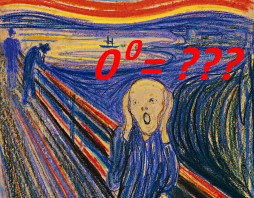Oh, the ambiguity!Introduction

Most of us learned in high school that 00 is somehow undefined or ambiguous. In college or university, your calculus professor will confirm this, citing the ambiguity resulting from different limits. We have Lim (x à 0): x0 = 1. But we also have Lim (y à 0): 0y = 0. There is an obvious discontinuity in the function z = xy at (0, 0). (See 00 with Continuous Exponents)

Your algebra professor, on the other hand, may tell you that you can assume that 00 = 1 on the natural numbers--for convenience mostly. They may justify it with analogies to various conventions, e.g. usually the convention of so-called empty products--the product of no numbers? Many simply define it to be 1. It’s apparently not something you can actually prove. As for 00 being undefined on the real numbers, and exponentiation being entirely consistent in both domains, that is a mere coincidence. We are talking about entirely different functions here, they will say. If that strikes you as being just a bit too, well, “hand wavy” for your liking, read on!

Here, I will develop the exponentiation function on the natural numbers with 00 undefined, given only the operations of addition and multiplication on N. I use what I believe to be a novel approach that looks at all possible functions that satisfy the usual requirements for an exponentiation function on N. In so doing, we can justify leaving 00 undefined, as it is on the set of real numbers R. I will also look at some implications for the usual laws of exponents on N for undefined 00.

Exponentiation Defined as Repeated Multiplication on N

When you were first introduced to exponents in elementary or high school, you probably started with the exponents greater than or equal to two. After all, you need at least 2 numbers to multiply. For all a ε N, we have:

a2 = a.a

a3 = a.a.a = a2. a

a4 = a.a.a.a = a3. a

a5 = a.a.a.a.a = a4. a

and so on.

This infinite sequence of equations can be recursively summarized in just two equations for all a, b ε N as follows:

1. a2 = a. a

2. ab+1 = ab. a

These two equations, by themselves, do not, however, tell us anything about exponents 0 or 1. It turns out that there are infinitely many such exponent-like functions on N that satisfy these equations.    Proof (21 lines)

Fortunately, these infinitely many functions differ only in the value assigned to 00.  Proof (194 lines)

This suggests that, in our definition of exponentiation on N, we should simply leave 00 undefined. To this end, we can construct (i.e. prove the existence of) a unique partial function for exponentiation on N.   Proof (618 lines)

Thus we can define exponentiation on N as follows:

1.     ab ε N   (for a or b ≠ 0)

2.     01 = 0

3.     a0 = 1    (for a ≠ 0)

4. ab+1 = ab. a    (for a or b ≠ 0)

The Laws of Exponents on N for undefined 00

Using the above definition, we can derive the 3 Laws of Exponents on N:

1.     The Product of Powers Rule:     ab. ac = ab+c     (for a ≠ 0  OR  both b, c ≠ 0)           Proof

2.     The Power of a Power Rule:      (ab)c = ab.c        (for a ≠ 0  OR  both b, c ≠ 0)           Proof

3.     The Power of a Product Rule:   (a.b)c = ac. bc   (for c ≠ 0  OR  both a, b ≠ 0)           Proof

Interestingly, these restrictions would not apply if 00 was defined to be either 0 or 1. So, adding these laws to the requirements for exponentiation would narrow down the infinitely many possibilities to only two. But we would still be left with some ambiguity—is 00 equal to 0 or 1? Oh, the ambiguity!

My Recommendation

If, as in most well used, centuries-old textbook applications where an informal proof will usually suffice, it is convenient and probably safe to assume 00=1 on the natural numbers, if not on the reals. If, however, you are pushing the limits of number theory and only a formal proof will do, you should take the extra care and avoid assuming any particular value for 00 in both the real numbers and the natural numbers. There are easy work-arounds in many cases. Applications of the binomial theorem to obtain the usual expansions of (x+y)n, for example, will result in terms including 00 when either x or y are zero. In such cases, however, there is a simple work-around, e.g. (x+0)n = xn for x or n  ≠ 0.